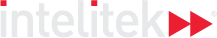### Course Overview

Algebra 2 covers linear and compound inequalities, including how to represent them graphically. We then proceed to working with systems of linear equations with two variables, as well as equations with no solution.

HOURS OF INSTRUCTION: 15

### Course Outline

• Solving Linear and Compound Inequalities
• Graphing Linear Equations and Inequalities
• Systems of Linear Equations with Two Variables
• Systems of Linear Equations with Three Variables
• Word Problems